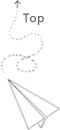# Python based non linear regression

Some tips about how to use the linear or non-linear regression approach in python.

### Mathematical background

For these kind of algorithm, we do not need to know all details about the math background since we mainly library provided by others instead of implementing it from the scratch.

This video provides some detailed background about how this works. The main idea is to come up with a expression about residual and then a matrix vector multiplication question. The metrix is jacobian matrix, the vector is the change of the parameters used that defines the non-linear curve.

### python program

The scipy provids a good abstraction about the linear/nonlinear least squares fitting this is an example:

https://github.com/wangzhezhe/5MCST/blob/master/python_scipy/least-square.py

In this example, we assume the x is the resource of a particular algorithm and the y is the execution time of that algorithm, we try to come up with a fitting function that describe how the execution time changes with different resources. For this simple case, the least_squares interface only need two parameters, the first one is a function that we provided to describe the residual (for each input parameters, we return its error compared with the actual data), the second one is the initial guess of the function. For the model function, it also requires two parameters, the first one is the list of variables used in the function, such as the theta in this case, the second one is the x.

In summary, we need three function rr_model that describe the fitting curve we provided, rr_fun use the rr_model and actual data to return the error. least_squares use the rr_fun and initial guess to return the predicted results. Then we use the predicted parameters and the the rr_model to compute the predicted results, we can compare the predicted results with the actual data to evaluate the error of the model prediction.

### Others

There are some practical techniques, such as taking the log operator of the raw data or how to select a good fitting curve. These things are more depedent on the actual experiences.

When we get a fitting function and assocaited values used in the function, we usually plot several extra steps and to see if the trend match the tren of the data.

Personally speaking, these fitting functions based on least square more suitable for the data which have a simple shape of the curve. It is pure statistical approach and the term in the fitting function might be meaningless from the aspect of the real problem. (how every term contributes to the results in a real problem). This blog describes some assumptions about using the linear least square approach. (It is important to figure out the assumption before adopting a particular approach)

Be careful of the difference between the curve fitting and the interpolation, for the curve fitting, we use known points to generate a curve and then use the curve (predicted model) to predict the point with a different location. For the interpolation, we use the known points to compute unknonwn points. Assuming the value of the unknown point is P, its value is P = w1*P1+w2*P2+...wn*Pn and the P1, P2 … Pn are known points.

### Reference

https://hernandis.me/2020/04/05/three-examples-of-nonlinear-least-squares-fitting-in-python-with-scipy.html

Math background of nonlinear least squares